2022: SklogWiki celebrates 15 years on-line

Melting curve

Melting curve

Empirical "one-phase" rules

Ross melting rule

The Ross melting rule states (Eq. 4 ):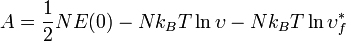$A = \frac{1}{2} NE(0) - Nk_BT \ln \upsilon - Nk_BT \ln \upsilon_f^*$

where$A$ is the Helmholtz energy function,$N$ is the number of cells,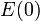$E(0)$ is the potential at the centre of the cell,$k_B$ is the Boltzmann constant,$T$ is the temperature,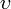$\upsilon$ is the volume of the cell, and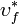$\upsilon_f^*$ is the dimensionless reduced volume in configuration space.

Khrapak melting criteria

The Khrapak one-phase melting criteria for two dimensional crystals with soft long-ranged interactions is given by (Eq 3 in ):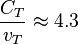$\frac{C_T}{v_T}\approx 4.3$

where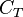$C_T$ is the transverse sound velocity, and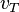$v_T$ is the thermal velocity.